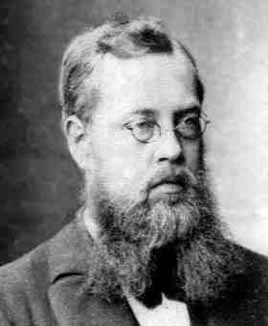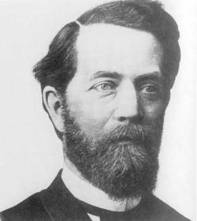# Research interestsThe simplest examples of Lie groups are groups of matrices that students already encounter in the basic courses on Linear Algebra. One research focus of the group are infinite dimensional Lie groups, which are generalizations of matrix groups. These are groups carrying a smooth manifold structure for which the group operations are smooth. A natural first order approximation of a Lie group is its Lie algebra and the translation mechanism between Lie groups and Lie algebra lies at the heart of Lie theory. This is analogous to modeling a dynamical system by a differential equation (infinitesimal level) and to recover the dynamics (global level) by an integration process.

The representation theory of Lie groups and their Lie algebras deals with the different realizations of a Lie group by symmetries of a mathematical structure and plays an important role in classifying objects in terms of their symmetries. Felix Klein‘s Erlangen program even defines geometry in terms of the corresponding symmetry groups. Lie groups and their Lie algebras also play a fundamental role as symmetries of classical and quantum mechanical systems. Here classical systems are modeled by Hamiltonian Lie group actions and quantum systems lead to unitary representations of Lie groups, an area which is under active investigation for infinite dimensional groups.

A particularly nice example of this correspondence is the situation where the classical system is described by Poisson-Lie groups – Lie groups that are at the same time Poisson manifolds in such a way that the Poisson structure and the Lie group multiplication are compatible. In this case, the corresponding quantum systems no longer exhibit Lie group symmetries but are gouverned by quantum groups.

Quantum groups are associative unital algebras with additional structure which ensures that their representation theory behaves similar to that of groups. The representation theory of quantum groups exhibits very rich mathematical features and can be generalised to an abstract formulation in terms of categories. What makes it particularly interesting is that it connects various aspects from algebrageometry and topology. Important examples are the well-known relation between the representation theory of quantum groups and knot theory as well as quantum manifold invariants constructed from spherical or ribbon categories.

Quantum group symmetries also play an important role in many areas of mathematical physics. They arise in the quantisation of integrable systems, in the description of the fractional quantum Hall effect, in the quantisation of Chern-Simons gauge theory, in non-commutative geometry as well as in quantum geometry and quantum gravity.